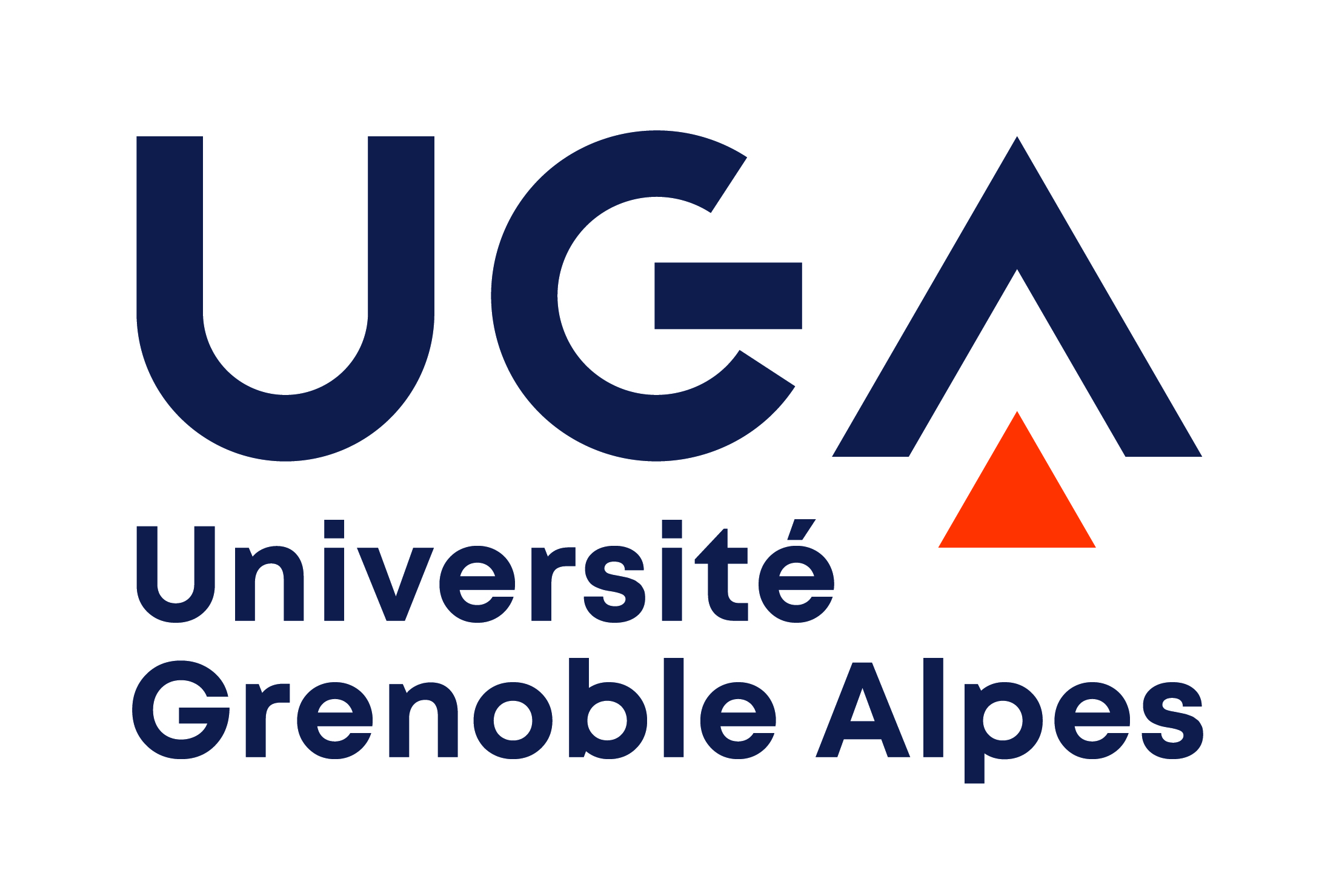# bibtex

@inproceedings{GM11,
title = { Improving Strategies via {SMT} Solving },
author = {Gawlitza, Thomas and Monniaux, David},
year = {2011},
booktitle = {ESOP},
team = {SYNC,PACSS},
abstract = {We consider the problem of computing numerical invariants of programs by abstract interpretation. Our method eschews two traditional sources of imprecision: (i) the use of widening operators for enforcing convergence within a finite number of iterations (ii) the use of merge operations (often, convex hulls) at the merge points of the control flow graph. It instead computes the least inductive invariant expressible in the domain at a restricted set of program points, and analyzes the rest of the code en bloc. We emphasize that we compute this inductive invariant precisely. For that we extend the strategy improvement algorithm of [Gawlitza and Seidl, 2007]. If we applied their method directly, we would have to solve an exponentially sized system of abstract semantic equations, resulting in memory exhaustion. Instead, we keep the system implicit and discover strategy improvements using SAT modulo real linear arithmetic (SMT). For evaluating strategies we use linear programming. Our algorithm has low polynomial space complexity and performs for contrived examples in the worst case exponentially many strategy improvement steps; this is unsurprising, since we show that the associated abstract reachability problem is Pi-p-2-complete. },
}

PDF